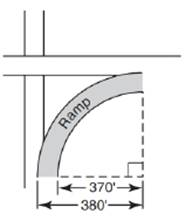Chapter 8.5, Problem 43EElementary Geometry For College St...

7th Edition
Alexander + 2 others
ISBN: 9781337614085

Solutions

Chapter
SectionElementary Geometry For College St...

7th Edition
Alexander + 2 others
ISBN: 9781337614085
Textbook Problem

An exit ramp from one freeway to another freeway forms a 900 arc of a circle. The ramp is scheduled for resurfacing. As shown, its inside radius is 370 ft, and its outside radius is 380 ft. What is the area of the ramp to the nearest integer?To determine

To find:

The area of the ramp to the nearest integer.

Explanation

Formula:

Area of sector:

If r is the radius of the circle, the area A of a sector whose arc has degree measure m is given by

A=m360×πr2.

Calculation:

To find the area of the ramp, we have to subtract the area of inner sector with radius 370 ft. from the area of outer sector with radius 380 ft.

Area of the ramp =Aouter sector-Ainner sector

It is given that the ramp forms an arc of 900.

Let’s find the area of outer sector.

From the diagram, it is clear that the radius of outer sector is 380 ft.

Let’s substitute the value of radius (380 ft) of outer sector and m=900 to find the area of outer sector.

Asector=m360×πr2

Aouter sector=90360×π×3802

Simplify:Aouter sector=14×π×144400

Simplify: Aouter sector=36100π ft2

Still sussing out bartleby?

Check out a sample textbook solution.

See a sample solution

The Solution to Your Study Problems

Bartleby provides explanations to thousands of textbook problems written by our experts, many with advanced degrees!

Get Started

What is the defining characteristic of a repeated-measures or within-subjects research design?

Essentials of Statistics for The Behavioral Sciences (MindTap Course List)

In Exercises 73-80, find the indicated limits, if they exist. 79. limxx5x3+x1x6+2x2+1

Applied Calculus for the Managerial, Life, and Social Sciences: A Brief Approach

The area of the shaded region is given by:

Study Guide for Stewart's Single Variable Calculus: Early Transcendentals, 8th